# How Many BTUs Will You Need To Cool A Room With An AC?

Need to calculate how many BTUs you will need to cool a room with an air conditioner? Finding out or calculating how many BTUs you need to properly cool a room with a Portable AC or Window Air Conditioner is critical. To properly cool a room with any type of air conditioner, you need to know the square footage of the room you are going to cool. Use the chart below to find out EXACTLY how many BTUs you need to air condition or cool a room.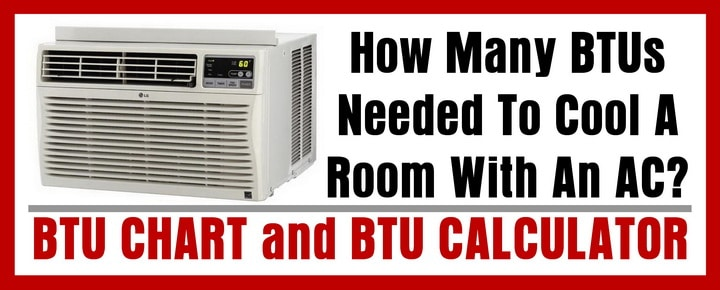The first thing you need to do is determine the square footage of the room you are going to cool. Measure the room’s LENGTH X WIDTH and you will have the square footage of the room. Once you have the square footage of the room, you can use the chart below to find out how many BTUs will be needed to cool the room properly.

Find the square feet (area being cooled) and then match the square footage to BTUs per hour (capacity needed).

## Square Footage to BTUs Needed Chart:

Room To Be Cooled (square feet) = 100 to 150
Capacity Needed (BTUs per hour) = 5,000

Room To Be Cooled (square feet) = 150 to 250
Capacity Needed (BTUs per hour) = 6,000

Room To Be Cooled (square feet) = 250 to 300
Capacity Needed (BTUs per hour) = 7,000

Room To Be Cooled (square feet) = 300 to 350
Capacity Needed (BTUs per hour) = 8,000

Room To Be Cooled (square feet) = 350 to 400
Capacity Needed (BTUs per hour) = 9,000

Room To Be Cooled (square feet) = 400 to 450
Capacity Needed (BTUs per hour) = 10,000

Room To Be Cooled (square feet) = 450 to 550
Capacity Needed (BTUs per hour) = 12,000

Room To Be Cooled (square feet) = 550 to 700
Capacity Needed (BTUs per hour) = 14,000

Room To Be Cooled (square feet) = 700 to 1,000
Capacity Needed (BTUs per hour) = 18,000

Room To Be Cooled (square feet) = 1,000 to 1,200
Capacity Needed (BTUs per hour) = 21,000

Room To Be Cooled (square feet) = 1,200 to 1,400
Capacity Needed (BTUs per hour) = 23,000

Room To Be Cooled (square feet) = 1,400 to 1,500
Capacity Needed (BTUs per hour) = 24,000

Room To Be Cooled (square feet) = 1,500 to 2,000
Capacity Needed (BTUs per hour) = 30,000

Room To Be Cooled (square feet) = 2,000 to 2,500
Capacity Needed (BTUs per hour) = 34,000

BTU Calculator
The BTU Calculator estimates the amount of BTUs you need to heat or cool your home based on the size and the temperature you want for your home.BTU chart for room size – Air Conditioning – AC

Note that the BTU chart does not know how hot it gets where you live. The BTU chart values assume that the AC in your room will be set at 72 degrees F. The chart is expressing the maximum cooling ability of the unit. The chart gives you recommendations for a 100% duty cycle which means the AC unit is ON all the time. See below for other factors to consider when calculating BTUs to cool a room.

Here are some other factors you need to take into consideration when figuring out BTUs for a room:
Are there sources of heat in the room, such as a fridge, TV, entertainment centers, etc?
Are there large windows in the room?
Will the AC unit be located in the center of the room, near a corner, or in the window?
How many exterior doors are in the room?
Is the room or area insulated?
How many walls in the room are exterior walls and facing the sun?

No BTU chart or formula can give an exact BTU rating required for an area or room. A room or area needing cooled may have other factors involved. Things such as large windows, bad insulation, and walls that face the exterior and exposed to the sun can make the room hotter. Because of these factors, you can choose an AC unit that produces more cooling. In these circumstances, you can go up 30% or more to get maximum cooling for the area needed. Do not go too high up on the BTUs as it is possible to have too much cooling for a given area and an air conditioning unit can freeze up.

## What does BTU stand for and the meaning?

BTU refers to the units of measure = British Thermal Units. The British Thermal Unit, or BTU, is an energy unit. It is the energy needed to heat one pound of water for 1 Fahrenheit. This is the measure for the cooling and heating of a room.
1 BTU = 1,055 joules
1 BTU per hour = 0.293 watts

An example of square footage to BTU is:
A 1,000 square foot home with proper insulation in Ohio needs a 20,000 to 25,000 BTU air conditioner for proper cooling in the summer. If there are factors such as the sun hitting the home on the roof and walls all day, large windows in the home, the efficiency of the home’s insulation, things in the home creating heat such as a refrigerator, dishwasher, computers, etc… These factors will change the home on an individual basis and a higher BTU may be needed.

Below are examples of Window mounted Air Conditioners and Portable Air Conditioners with different BTU ratings. The range of the BTUs goes from 5,000 BTUs to 36,000 BTUs, which is comparable with the chart above.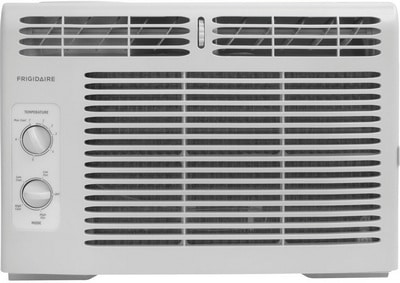5000 BTU Window Mounted Mini Compact Air Conditioner8000 BTU Window Mounted Air Conditioner10000 BTU Window Mount Air Conditioner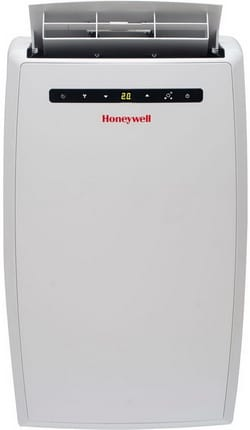10000 BTU Portable Air Conditioner12000 BTU Window Mounted Air Conditioner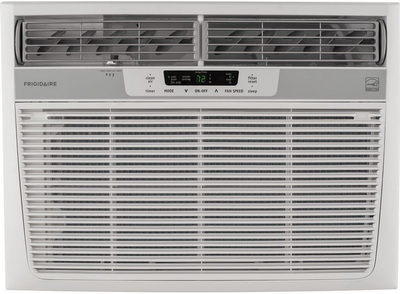25000 BTU Window Mount Heavy Duty Air Conditioner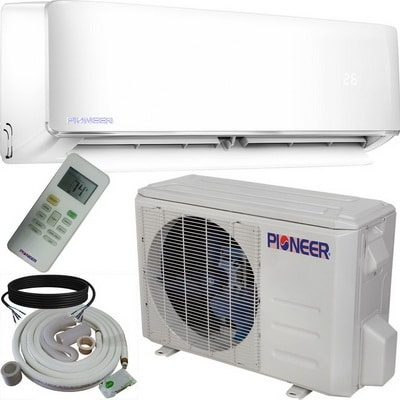36000 BTU Air Conditioner Inverter Ductless Wall Mount Mini Split System

Need help determining how many BTUs you need to cool a room with an air conditioner? Please leave your question below and we will be more than happy to assist you.